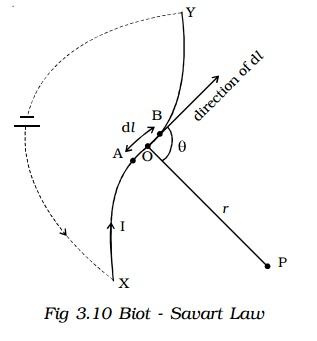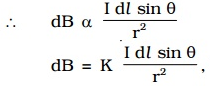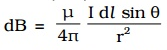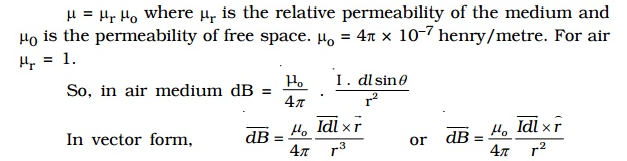Biot and Savart conducted many experiments to determine the factors on which the magnetic field due to the current in a conductor depends.The results of the experiments are summarized as Biot-Savart law.

Let us consider a conductor XY carrying a current I (Fig 3.10). AB = dl is a small element of the conductor. P is a point at a distance r from the midpoint O of AB. According to Biot and Savart, the magnetic induction dB at P due to the element of length dl is

1. directly proportional to the current (I)
2. directly proportional to the length of the element (dl )
3. directly proportional to the sine of the angle between dl and the line joining element dl and the point P (sin θ)
4. inversely proportional to the square of the distance of the point from the element (1/r2)K is the constant of proportionality

The constant K = μ/4π where μ is the permeability of the medium.The direction of dB is perpendicular to the plane containing current element Idl and r (i.e plane of the paper) and acts inwards. The unit of magnetic induction is tesla (or) weber m-2.